# Numb3rs 322: Under Pressure

In this episode, Charlie talks about structures of graphs and social networks as well as applying probability to determine likely properties of social networks. In what follows, we will describe various basic properties of graph structure as well as give some techniques used in network theory.

## Return times

Consider the integer points on the real number line. Between each pair of consecutive integers, put an edge. We'll consider this as a long street with each integer point as a house on the street. Suppose you start at your house (we'll call it zero). You go left with probability 1/2 and right with probability 1/2 and stop at the next house. Again, you will go left with probability 1/2 or right with probability 1/2, and so on.

### Activity 1:

Let's try to answer this problem. Let Sn denote our position after n steps. Let P(Sn=0) denote the probability that Sn is zero. Obviously, to get back to zero, we have to make exactly as many right steps as we do left steps. So, it must be the case that P(Sn=0)=0 whenever n is odd. Thus we consider only P(S2n=0). Now we are left with a counting problem:

### Activity 2:

Suppose we have 2n boxes, n red balls, and n blue balls. Show that there are (2n)!/(n!)2 ways of putting the balls into the boxes.
Since each path has probability (1/2)2n of occuring, we see that P(S2n=0)=(1/2)2n × (2n)!/(n!)2. This means thatThis gives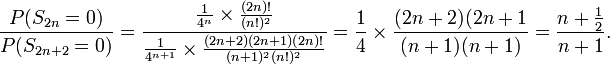Multiplication givesso that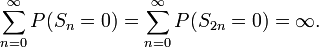Let T0=0. Define T1 to be the first time at which a walk hits zero, T2 to be the second time a walk hits zero, and so forth. T1 is called the first return time. I'm sure you can guess what T2 is called!

### Activity 3:

Convince yourself that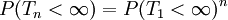This probability with infinity in it is the probability that our walk hits zero at least n times. To prove this rigorously, you will probably need to read about conditional probability, Bayes's Theorem, and independent events.

### Activity 4:

Convince yourself that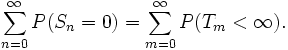Basically, the left hand side "counts" the number of paths which hit zero after 0, 1, 2, etc. steps. The right hand side "counts" how many paths hit zero 0 once in finite time, twice in finite time, and so forth. So really they're counting the same things.

If x is a positive number smaller than 1, thenis a well defined function. Assuming that we can legitimately multiply infinite sums how we would like to, we see that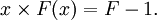Hence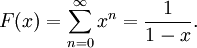This means that if P(T1) < 1, then the equation in Activity 3 implies that the right hand side of the equation in Activity 4 is finite. However, we showed earlier that the left side of the equation in Activity 4 is infinite. So it must be the case that P(T1) = 1. What we have just shown is that if we randomly walk left and right, we will return home with certainty! Phew!

One could ask the same question in the scenario of the integer lattice on the plane (i.e. vertices at with integer coordinates and edges drawn between the four closest vertices to each point). In this scenario we have 1/4 probability of going up, down, left, or right. With regards to returning home, it turns out that after computing P(S2n = 0) and doing some algebra the above argument is enough to answer the question affirmatively. However, if we do the same thing on a 3 dimensional grid of points in space with 1/8 probability of going in any direction, the probability of returning to the same point twice is ZERO! So you can find your way home with your eyes closed, and yet a a blinded bird would not be so lucky!

## Kolmogorov's 0-1 Law

Kolmogorov (1903-1987) was a mathematician from the former Soviet Union. Among a number of major accomplishments in his career, he revolutionized the study of probability. He falls into a mathematical pedigree (where one thinks of their adviser as their mother or father) of superstars from the field of analysis including Carl Gauss, Karl Weierstrass, Dimitri Egorov, and Nikolai Luzin.

Kolmogorov's 0-1 Law is a very general statement, but in many cases the statement is completely obvious. The idea is as follows. Suppose we have an infinitely long sequence of independent experiments (the probabilistic term is a sequence of independent random variables). Consider an event which is independent of any finite number of the first experiments. Such an event is called a tail event because it is basically unaffected by the first n terms for any n. Kolmogorov's 0-1 Law says that any tail event has either probability 0 or 1 but no value in between!

Suppose we have a sequence of independent experiments which randomly output an integer 0 through 9. We could ask the question of what the probability of the sequence converging is. That is, with what probability should we expect the sequence to have an infinite string of 0s,1s,2s, etc. Now obviously this has probability zero since the probability of a string of any digit of length n is (1\10)n which tends to zero for large n. The Kolmogorov 0-1 Law tells us that this probability is either 0 or 1, though it does not tell us which one it is.

Before you come to the conclusion that the above example is uninteresting, consider what its implication is.

### Activity 5:

What is the probability of obtaining a rational number when selecting a real number at random between 0 and 1? Here "at random" means that the probability of picking a real number x with a < x < b is just b-a and does not depend on the position of a or b.

Even though the Kolmogorov 0-1 Law gives only two possible probabilities for tail events, it is often incredibly difficult to determine whether a particular event has probability 0 or 1. The following discussion elaborates on this.

## Random Graphs and Percolation

We again consider the integer points of the plane. Now, instead of putting an edge between nearest neighbors, we do something slightly different. With probability p (some number between 0 and 1) we put an edge between two nearest neighbors and don't put an edge between them with probability 1-p. This will construct a subgraph of the integer lattice with some degree of randomness, so the term random graph seems appropriate (although a general random graph need not be a subgraph of the integer lattice).

We can ask whether or not there is an infinitely long path in this randomly drawn graph. The Kolmogorov 0-1 Law says that this happens either with probability 0 or probability 1. For p=0, this must have probability 0 since we never draw any edges at all. For p=1, this must have probability 1 since we draw the integer lattice. So for some value pc, we find that there no infinitely long paths for values strictly less than pc and there are always infinitely long paths for values strictly greater than pc. The particular value pc is on the boundary between the two, so a separate argument would be required to determine whether there are or are not infinitely long paths at p = pc. The value pc is called the critical probability. The set smaller values of p is called the subcritical phase, and the set of larger values of p is called the supercritical phase.

That's all well and good, but what is pc? For any particular value of p, we know with probability 0 or 1 that there is a path of infinitely long length. However, it is very difficult to determine which it is! For the question in the plane, it is known that pc=1\2. However, the value of pc is not known for the analogous question in 3 dimensions.

For a longer description of percolation, Harry Kesten - a former professor of Cornell University - wrote an article in the Notices of the AMS describing percolation. You can find it here.